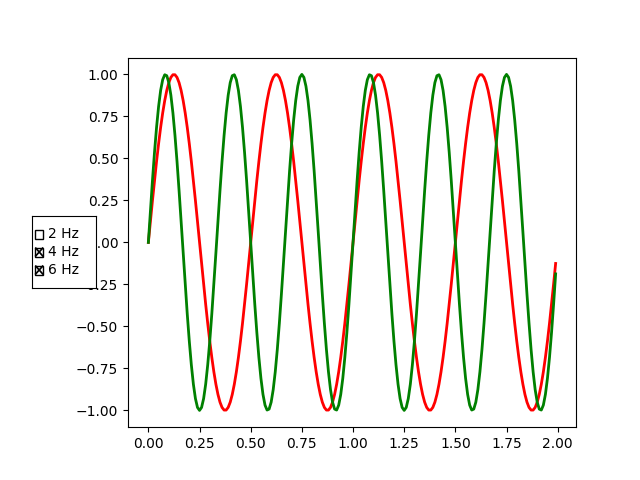# Check Buttons¶

Turning visual elements on and off with check buttons.

This program shows the use of ‘Check Buttons’ which is similar to check boxes. There are 3 different sine waves shown and we can choose which waves are displayed with the check buttons.```import numpy as np
import matplotlib.pyplot as plt
from matplotlib.widgets import CheckButtons

t = np.arange(0.0, 2.0, 0.01)
s0 = np.sin(2*np.pi*t)
s1 = np.sin(4*np.pi*t)
s2 = np.sin(6*np.pi*t)

fig, ax = plt.subplots()
l0, = ax.plot(t, s0, visible=False, lw=2, color='k', label='2 Hz')
l1, = ax.plot(t, s1, lw=2, color='r', label='4 Hz')
l2, = ax.plot(t, s2, lw=2, color='g', label='6 Hz')

lines = [l0, l1, l2]

# Make checkbuttons with all plotted lines with correct visibility
rax = plt.axes([0.05, 0.4, 0.1, 0.15])
labels = [str(line.get_label()) for line in lines]
visibility = [line.get_visible() for line in lines]
check = CheckButtons(rax, labels, visibility)

def func(label):
index = labels.index(label)
lines[index].set_visible(not lines[index].get_visible())
plt.draw()

check.on_clicked(func)

plt.show()
```

Gallery generated by Sphinx-Gallery